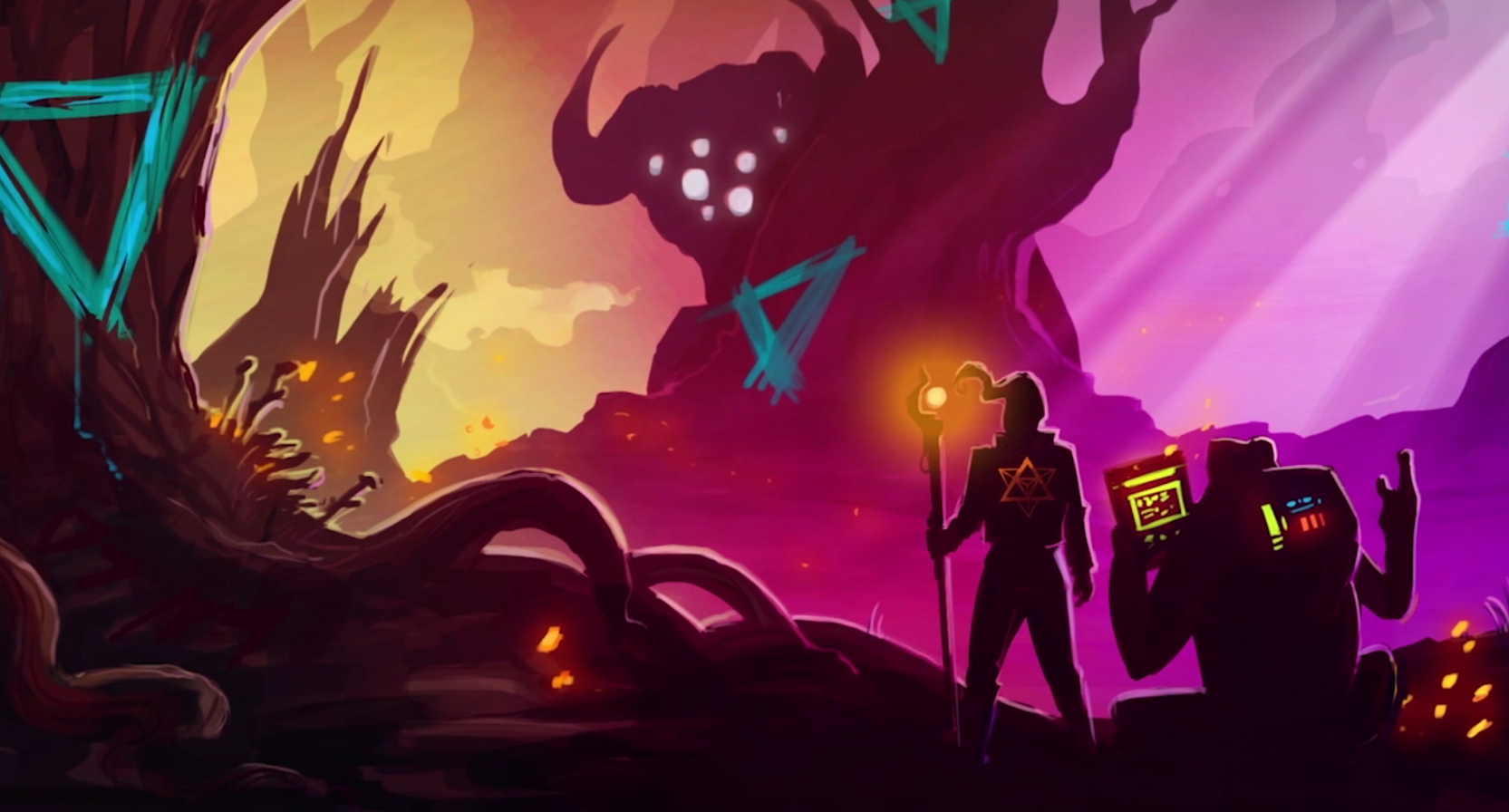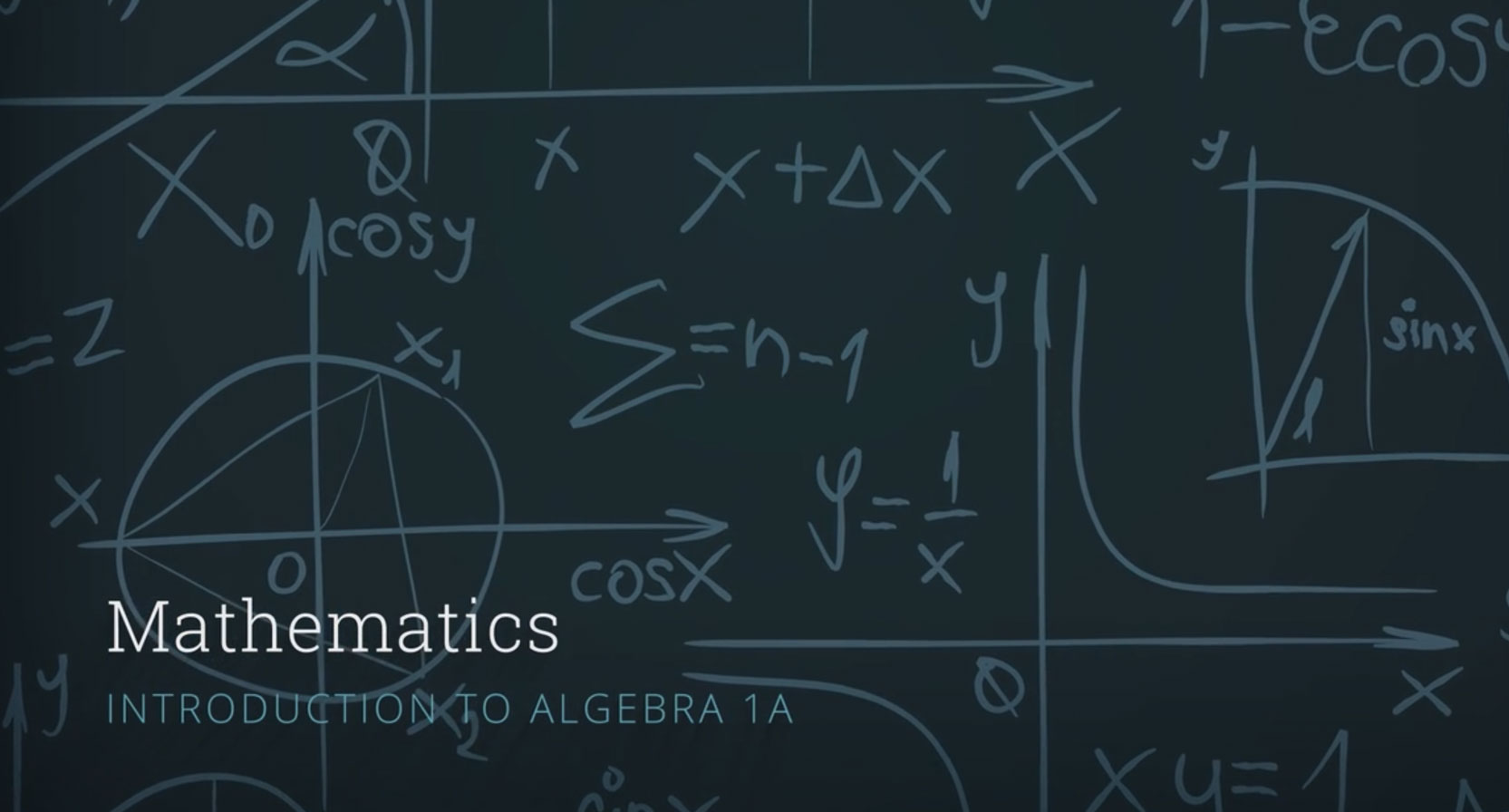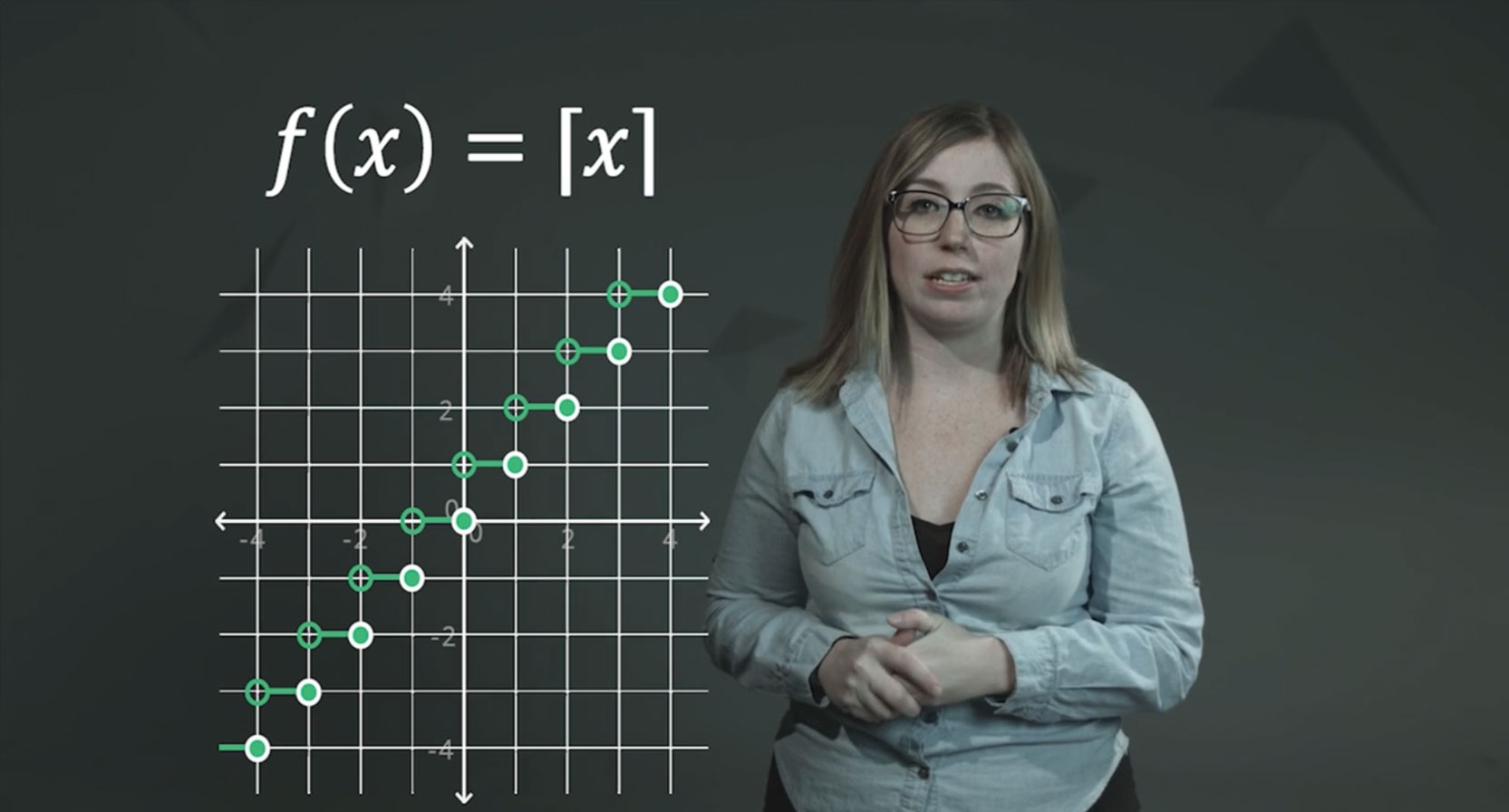# HIGH SCHOOL: MATHEMATICS

Curriculum for the Digital Generation

## HIGH SCHOOL : MATHEMATICS

Utilizing an innovative math curriculum, students master concepts in subject areas such as Algebra, Geometry, and Advanced Math. This interactive format is challenging, yet engaging, and exceeds the most demanding academic expectations.

93%

Highly Satisfied

Guaranteed

Satisfaction

Award

Winning

Learner

Centered Design

Outcomes

Based

State Aligned

Designed

## COURSE INTRO VIDEOS

Geometry AGeometry BAlgebra 1AAlgebra 1BAlgebra 2AAlgebra 2B## COURSE CATALOG

#### ALGEBRA 1A

Algebra 1A helps students build a strong foundation of traditional algebra concepts. Students will delve deep into algebraic problems and apply their knowledge to real-life situations.

In this course, students learn the properties of different forms of linear equations and inequalities and their graphs. Students also learn how to define a function and how to relate linear equations and functions. Students will solve systems of equations and systems of inequalities and interpret their solutions mathematically and contextually. The course concludes with a study of statistics, which helps students to discover some of the interesting ways that math is used to describe the world.#### ALGEBRA 1B

Algebra 1B extends students’ algebraic skills and knowledge to five new types of functions: quadratic, exponential, absolute-value, piecewise, and step.

In this course, students will learn the properties and key features of nonlinear functions, including quadratic functions and exponential functions. Students will identify key features and interpret functions presented as equations, graphs, tables, and verbal descriptions. Emphasis will be placed on how different families of functions compare to one another, and transformations of functions will be performed for all function types presented. The course will conclude with an introduction of “other” functions, including absolute-value functions, piecewise functions, and step functions.#### GEOMETRY A

The content in each lesson of Geometry A is interwoven, in a story-telling style, with the adventures of Geomethor, a superhero who strives to save his world using Geometry.

Students in this course will develop a deep understanding of the logical and rigorous proving system of geometry. Students will begin by looking at congruence, proofs, and constructions. In doing so, they define and use the basic geometric terms, then advance to proving statements about lines, angles, triangles, and quadrilaterals. Students apply the knowledge they have of planar transformations to learn a formal definition for similarity, and then they use that definition to write proofs and construct figures.

In addition, students are introduced to trigonometry through its connection to the concept of similarity. Finally, students will explore three-dimensional figures such as planar solids and solids of revolution. Students can apply the basic understanding of geometric figures that they achieved in middle school mathematics to a more formal, rigorous proof system. Students also incorporate algebraic concepts into the same formal proof methodology. In doing so, students can better prepare themselves for the complexity of Algebra 2 and have a basis for understanding the concepts of calculus and advanced mathematics.#### GEOMETRY B

In this course, students will use the Pythagorean theorem, distance formula, midpoint formula and slope formula to solve geometric problems and develop coordinate proofs.

They will connect the algebraic and geometric meaning of a parabola by using the distance formula. Students will learn theorems about circles, find arc lengths and areas of sectors of circles, and use the distance formula to write equations of circles in the coordinate system. Students will also learn about counting methods to find the number of possible combinations of a group of objects. They will interpret theoretical and experimental probabilities, and use probability to analyze fairness of decisions. The course concludes with independent and dependent events, conditional probability, and mutually exclusive and inclusive events.#### ALGEBRA 2A

From construction to physics, the concepts in this Algebra 2 course are used in a variety of real-world situations.

In Algebra 2A, students will extend the knowledge of trigonometry that they gleaned from Geometry as well as build upon the algebra they learned in Algebra 1 to start modeling tons of real-world scenarios. Students learn that the polynomials and other expressions and equations that make up Algebra 2 are the basis for things they love: from video games, to roller coasters, to the home they live in, it all has a little algebra in it.#### ALGEBRA 2B

Algebra 2B completes the studies of high school algebra.

Students will be prepared to advance to trigonometry, pre-calculus, and beyond. Students will develop and strengthen skills to read and evaluate algebraic expressions. Topics covered include exponential and logarithmic functions, rational and radical functions, properties and attributes of functions, conic sections, and data analysis. It also includes an introduction to probability and trigonometry.#### PRE-ALGEBRA

Pre-Algebra is designed to give students the opportunity to build the conceptual understanding and skills necessary to be successful in Algebra 1A and Algebra 1B.

Students begin this course by reviewing operations with rational numbers. They find factors and multiples of numbers, along with common factors and multiples of sets of numbers. They also add, subtract, multiply, and divide integers, fractions, and mixed numbers. Students apply properties, such as the Distributive, Associative, and Commutative Properties, and use the order of operations to simplify numerical expressions and then algebraic expressions. Next, they work with algebraic equations and use them to solve problems. This is followed by identifying, graphing, and comparing linear relationships. The course concludes with an introduction to functions.